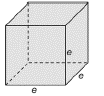Chapter 9.1, Problem 32E### Elementary Geometry for College St...

6th Edition
Daniel C. Alexander + 1 other
ISBN: 9781285195698

#### Solutions

Chapter
Section### Elementary Geometry for College St...

6th Edition
Daniel C. Alexander + 1 other
ISBN: 9781285195698
Textbook Problem
1 views

# Use the formulas and drawing in Exercise 31 to find ( a ) the total area T and (b) the volume V of a cube with edges of length 5.3 ft each.A cube is a right square prism in which all edges have the same length. For the cube with edge e , a ) Show that the total area is T = 6 e 2 . b ) find the total area if e = 4 cm. c ) Show that the volume is V = e 3 . d ) find the volume if e = 4 c m .To determine

a)

The total area T and.

Explanation

The total area of any solid is the area of all the number of lateral sides that it have, including the area of the top and the bottom of solid.

The volume of any solid is the region of space occupied by it and in general the volume of a solid is given by the product of its base area and altitude.

Calculation:

Given,

The cube of edge e=5.3 ft.

From exercise 31, the total area T of the cube is given by

T=6e2

But, we have e=5

To determine

b)

The volume V of a cube with edges of length 5.3 ft each

Using the formula and drawing in Exercise 31

### Still sussing out bartleby?

Check out a sample textbook solution.

See a sample solution

#### The Solution to Your Study Problems

Bartleby provides explanations to thousands of textbook problems written by our experts, many with advanced degrees!

Get Started

#### Multiply: 56748_

Elementary Technical Mathematics

#### If xy + ey = e, find the value of y at the point where x = 0.

Single Variable Calculus: Early Transcendentals, Volume I

#### In Exercises 7-28, perform the indicated operations and simplify each expression. 15. 4x295x26x+9

Applied Calculus for the Managerial, Life, and Social Sciences: A Brief Approach

#### 45 15 63 127

Study Guide for Stewart's Multivariable Calculus, 8th